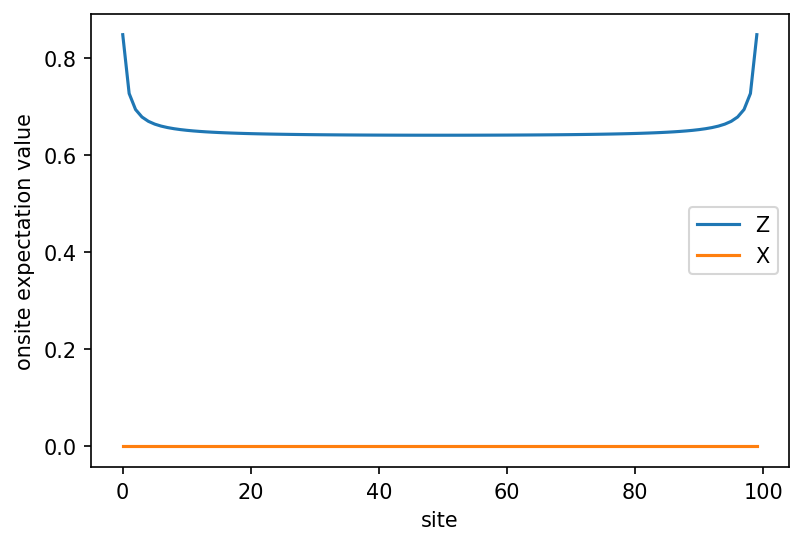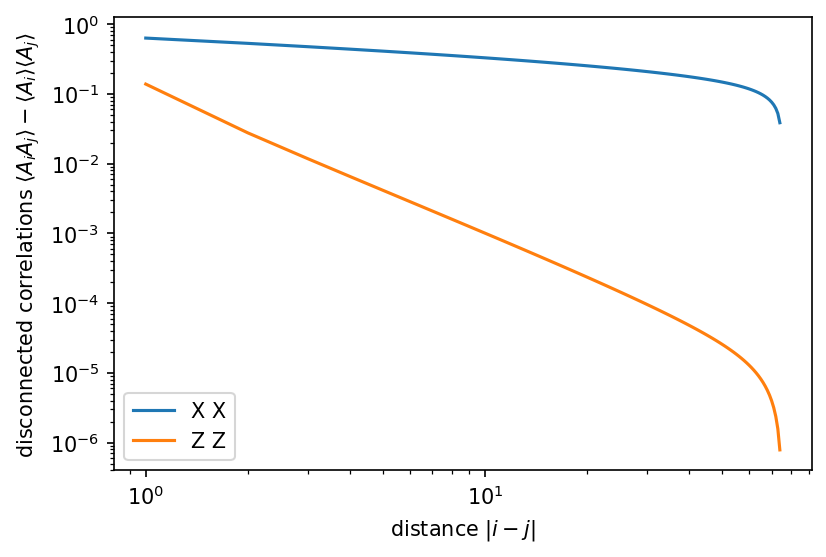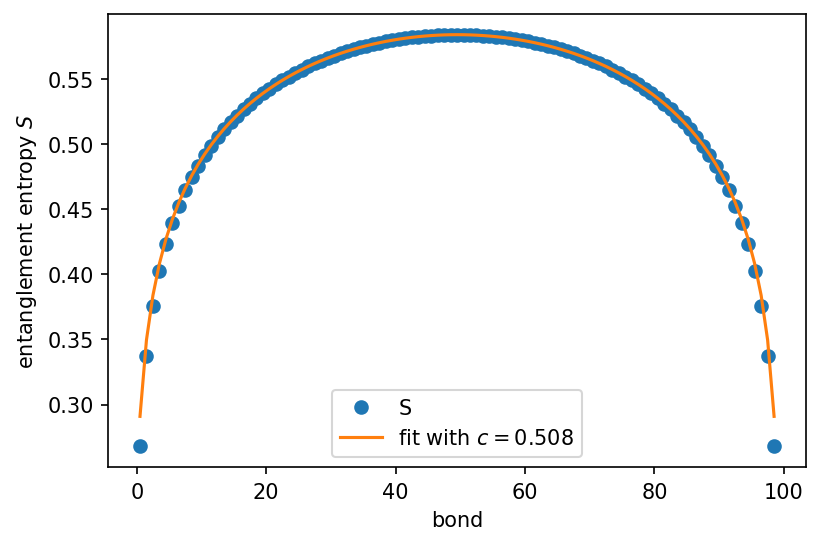# A first finite DMRG Example¶

Like examples/d_dmrg.py, this notebook shows the basic interface for DMRG. It initalized the transverse field Ising model $$H = J XX + g Z$$ at the critical point $$J=g=1$$, and a finite MPS in the all-up state $$|\uparrow\cdots \uparrow\rangle$$. It then runs DMRG to find the ground state. Finally, we look at the profile of the entanglement-cuts.

:

import numpy as np
import scipy
import matplotlib.pyplot as plt
np.set_printoptions(precision=5, suppress=True, linewidth=100)
plt.rcParams['figure.dpi'] = 150

:

import tenpy
import tenpy.linalg.np_conserved as npc
from tenpy.algorithms import dmrg
from tenpy.networks.mps import MPS
from tenpy.models.tf_ising import TFIChain

tenpy.tools.misc.setup_logging(to_stdout="INFO")

:

L = 100

:

model_params = {
'J': 1. , 'g': 1.,  # critical
'L': L,
'bc_MPS': 'finite',
}

M = TFIChain(model_params)

INFO    : TFIChain: reading 'bc_MPS'='finite'

:

psi = MPS.from_lat_product_state(M.lat, [['up']])

:

dmrg_params = {
'mixer': None,  # setting this to True helps to escape local minima
'max_E_err': 1.e-10,
'trunc_params': {
'chi_max': 100,
'svd_min': 1.e-10,
},
'verbose': True,
'combine': True
}
eng = dmrg.TwoSiteDMRGEngine(psi, M, dmrg_params)
E, psi = eng.run() # the main work; modifies psi in place

INFO    : TwoSiteDMRGEngine: subconfig 'trunc_params'=Config(<2 options>, 'trunc_params')
INFO    : Running sweep with optimization
INFO    : checkpoint after sweep 1
energy=-126.9290280127259649, max S=0.3829294433343728, age=100, norm_err=1.2e-01
Current memory usage 118.7MB, wall time: 3.0s
Delta E = nan, Delta S = 3.7668e-01 (per sweep)
max trunc_err = 0.0000e+00, max E_trunc = 1.4211e-13
chi: 4
================================================================================
INFO    : Running sweep with optimization
INFO    : checkpoint after sweep 2
energy=-126.9618018027223059, max S=0.5530509009591875, age=100, norm_err=7.0e-03
Current memory usage 122.5MB, wall time: 5.3s
Delta E = -3.2774e-02, Delta S = 1.3418e-01 (per sweep)
max trunc_err = 0.0000e+00, max E_trunc = 1.8474e-13
chi: 16
================================================================================
INFO    : Running sweep with optimization
INFO    : checkpoint after sweep 3
energy=-126.9618767384862963, max S=0.5837952098396202, age=100, norm_err=1.1e-05
Current memory usage 129.9MB, wall time: 7.5s
Delta E = -7.4936e-05, Delta S = 1.6908e-02 (per sweep)
max trunc_err = 4.9554e-20, max E_trunc = 2.1316e-13
chi: 64
================================================================================
INFO    : Running sweep with optimization
INFO    : checkpoint after sweep 4
energy=-126.9618767396805765, max S=0.5838739117880425, age=100, norm_err=5.2e-10
Current memory usage 138.0MB, wall time: 9.3s
Delta E = -1.1943e-09, Delta S = 3.5241e-05 (per sweep)
max trunc_err = 8.0041e-19, max E_trunc = 2.4158e-13
chi: 89
================================================================================
INFO    : Running sweep with optimization
INFO    : checkpoint after sweep 5
energy=-126.9618767396809176, max S=0.5838739119332658, age=100, norm_err=3.5e-13
Current memory usage 138.0MB, wall time: 4.0s
Delta E = -3.4106e-13, Delta S = 5.3635e-11 (per sweep)
max trunc_err = 6.8285e-20, max E_trunc = 2.2737e-13
chi: 90
================================================================================
INFO    : DMRG finished after 5 sweeps, max chi=90


## Expectation Values¶

:

# the ground state energy was directly returned by dmrg.run()
print("ground state energy = ", E)

# there are other ways to extract the energy from psi:
E1 = M.H_MPO.expectation_value(psi)  # based on the MPO
E2 = np.sum(M.bond_energies(psi))  # based on bond terms of H, works only for a NearestNeighborModel
assert abs(E-E1) < 1.e-10 and abs(E-E2) < 1.e-10

ground state energy =  -126.96187673968092

:

# onsite expectation values

X = psi.expectation_value("Sigmax")
Z = psi.expectation_value("Sigmaz")
x = np.arange(psi.L)
plt.figure()
plt.plot(x, Z, label="Z")
plt.plot(x, X, label="X")   # note: it's clear that this is zero due to charge conservation!
plt.xlabel("site")
plt.ylabel("onsite expectation value")
plt.legend()
plt.show():

# correlation functions

i0 = psi.L // 4  # for fixed i
j = np.arange(i0 + 1, psi.L)
XX = psi.term_correlation_function_right([("Sigmax", 0)], [("Sigmax", 0)], i_L=i0, j_R=j)
XX_disc = XX - X[i0] * X[j]
ZZ = psi.term_correlation_function_right([("Sigmaz", 0)], [("Sigmaz", 0)], i_L=i0, j_R=j)
ZZ_disc = ZZ - Z[i0] * Z[j]

dx = j - i0
plt.figure()
plt.plot(dx, XX_disc, label="X X")
plt.plot(dx, ZZ_disc, label="Z Z")
plt.xlabel(r"distance $|i-j|$")
plt.ylabel(r"disconnected correlations $\langle A_i A_j\rangle - \langle A_i \rangle\langle A_j\rangle$")
plt.legend()
plt.loglog()
plt.show()We find power-law decaying correlations, as expected for a critical model. For a gapped model, we would expect exponentially decaying correlations.

We now look at the entanglement entropy. The transverse-field Ising model is critical at $$g=J$$. Conformal field theory, Calabrese,Cardy 2004, predicts an entanglement entropy profile of

$S(l, L) = \frac{c}{6} \log\left(\frac{2L}{\pi a} \sin\left(\frac{\pi l}{L}\right)\right) + \textrm{const}$

where $$c=0.5$$ is the central charge, $$a$$ is the lattice spacing (we set $$a=1$$), $$L$$ is the total size of the system and we consider subsystems of size $$l$$ and $$L-l$$ as left/right. Note that this yields the familiar $$S(L/2, L) = \frac{c}{6} \log(L) + \textrm{const}$$ for the half-chain entropy as a function of system size.

:

S = psi.entanglement_entropy()

bonds = np.arange(0.5, psi.L-1)
plt.plot(bonds, S, 'o', label="S")

# preform fit to extract the central charge
central_charge, const, res = tenpy.tools.fit.central_charge_from_S_profile(psi)
fit = tenpy.tools.fit.entropy_profile_from_CFT(bonds + 0.5, psi.L, central_charge, const)
print(f"extraced central charge {central_charge:.5f} with residuum {res:.2e}")
print("(Expect central charge = 0.5 for the transverse field Ising model.)")
plt.plot(bonds, fit, label=f"fit with $c={central_charge:.3f}$")
plt.xlabel("bond")
plt.ylabel("entanglement entropy $S$")
plt.legend()
plt.show()

extraced central charge 0.50788 with residuum 1.95e-09
(Expect central charge = 0.5 for the transverse field Ising model.)[ ]: